#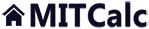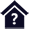Slender strut (column) buckling.

Clicking on an item from the content will take you to the relevant paragraph.

## Slender strut (column) buckling.

The program is designed to calculate the optimum cross-section and perform strength check of slender struts strained for buckling. The program includes:

• Selection of six basic types of buckling.
• Calculation of area characteristics of 20 types of cross-sections.
• Design of optimum profile accommodating the set load.
• Strength check of the strut.
• Calculation and graphical representation of permitted stress dependent on slenderness rate.
• A table of materials and a table of area characteristics of W, S, C and L profiles according to ANSI/AISC and I, U, L and T profiles according to DIN/ISO.

The calculation is based on data, procedures, algorithms (Johnson, Tetmajer, Euler, Secant) and data from specialized literature and AISC, ISO, DIN and BS standards.
List of standards (DIN 1025, 1026, 1028, 1029, 1024, AISC W, S, C, L, LU ... )

User interface.

Purchase, Price list.

## Control, structure and syntax of calculations.

Information on the syntax and control of the calculation can be found in the document "Control, structure and syntax of calculations".

## Information on the project.

Information on the purpose, use and control of the paragraph "Information on the project" can be found in the document  "Information on the project".

## Theory - Fundamentals.

Beams (struts) loaded by axial force are divided into three basic groups.

A.      Short beams (struts) –failure/deformation occurs when the yield point in compression is achieved. The beams are strained by simple compression. Critical force is calculated according to the following formula:where
sy...yield point
A...profile area

B.       Medium-length beams (struts) – are deformed in the area of inelastic buckling according to rather complicated relations and regularities. There are many theories and empirical methods dealing with calculation of critical force/stress; the most frequently used are:
- Linear compensation – mainly for tough metals
- Parabolic compensation – used for brittle materials

C.      Long beams (struts) –failure/deformation occurs significantly earlier than the stress exceeds permitted stress of the material. The failure appears as buckling and collapse of the strut. Long beams follow Euler’s formula in the zone of elastic buckling.where
E...Modulus of elasticity in tension
Leff...Reduced (effective) strut length

Comparison of individual theories (formulas) can be seen in the diagram of critical stress s dependent on slenderness rate of strut SR(l) Slenderness rate is a basic geometrical characteristic of the checked strut formulated by the formula: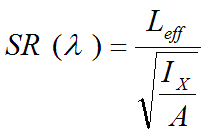where
Leff...Reduced (effective) strut length.
A...Profile area
Ix...Quadratic moment of inertia of profile

In the diagram, letters A,B and C determine areas of simple compression (A), nonelastic buckling (B) and elastic buckling (C).(The Secant formula has eccentricity degree set to 0.25 in this diagram)

#### Secant formula (method)

In cases when the force does not act directly in the strut axis (mounting imperfections) or if the strut is not accurately straight (inaccuracies in production, sag), the so-called Secant formula is used for calculation of stress in the extreme fibre of the profile.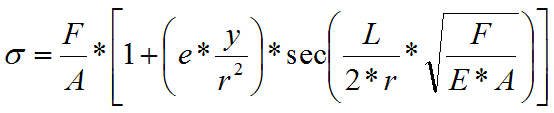where

F...Force
A...Profile area
E...Modulus of elasticity in tension
y...Extreme fibre distance
e...Deflection of origin of force or strut axis.

Equation in the brackets (e * y / r2) formulates the so-called eccentricity degree - m. With expected knowledge of m (estimation), the Secant formula is a very good substitution of the said empirical methods and is a basis for the whole range of suggested methods.

The diagram of critical stress s dependent on slenderness rate of the strut SR(l) shows curves for various values of eccentricity degree.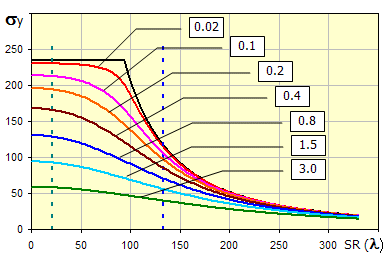## Process of calculation.

Calculation of optimum cross-section and strength check of slender struts includes the following steps:

1. Define the strut mounting type. [1.2]
2. Select profile type [2.2]
3. Select strut material (material properties). [2.11-2.14]
4. Set strut length and axial load. [3.1, 3.2]
5. Set safety coefficient and eccentricity degree. [3.6, 3.7]
6. Run the design of optimum strut cross-section using the "Start" button.
7. Check calculated values and save the workbook with the solution under a new name.

During the check, set not only the cross-section type, but also dimensional values of the profile you are checking and check relevant safety coefficients.

## Strut (column) mounting. 

In this paragraph, select units for calculation and the type of mounting of the strut stressed in buckling.

### 1.1 Calculation units.

Select the desired system of calculation units in the list box. After switching over the units, all values will be changed immediately.

### 1.2 Type of strut mounting.

In the drop down menu, select the type of strut mounting according to the picture. The selection of the type of mounting leads to selection of a coefficient of reduced (effective) length that is used to multiply the real beam length to achieve so-called reduced (effective) beam length used in calculations. Line [1.4] specifies the theoretical value of the coefficient; line [1.5] the engineering value recommended to be used in the calculation.

 Indication Strut mounting Coef.(theor) Coef.(pract) A Clamped - Clamped 0.50 0.65 B Clamped - Hinged 0.70 0.80 C Clamped - Guided 1.00 1.20 D Hinged - Hinged 1.00 1.00 E Clamped - Free end 2.00 2.10 F Hinged - Guided 2.00 2.00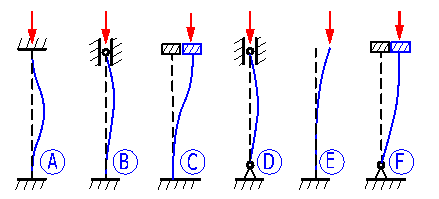### 1.6 Value used for calculation.

If the checkbox is checked, the value of the selected coefficient is put into the calculation, after the checkbox is unchecked, you can put in your own value of the coefficient of reduced (effective) strut length.

## Static values of the profile and material values. 

In this paragraph, select the type of beam, the respective static values and select a material.

### 2.2 Profile type.

Select the profile you want to use from the pop-up menu. Calculated profiles, selected rolled profiles according to ANSI/AISC and according to DIN are available. In the brackets following the name of the profile, there is a specification of the standard or indication of the calculated profile and a note on whether the value is a minimum or maximum of quadratic moment of inertia Ix.

After selection, the selected profile is shown in the illustration. In case you select a calculated profile, the input fields in which dimensions of the selected profile must be entered are shown to the right of the illustration. Follow the illustration when entering the dimensions.

### 2.3 Profile dimensions.

If you select a standardized profile, the dimensions range is available in the drop down menu. Select a suitable profile dimension.

### 2.4 User's parameters of the profile.

If the desired profile is not included in the database and calculated profiles also do not fit, your own static values of the profile can be entered. In such cases, switch over the selection to Yes and in the row [2.5,2.6 a 2.7] fill in the required values.

### 2.5, 2.6, 2.8 Static values of the profile.

The lines give static values of the selected profile. You can set your custom values using the switch in line 2.4.

### 2.7 Max. distance of fibre.

It is the distance of the extreme fibre from the profile axis which goes through the centre of gravity. This value is required for calculation using the "Secant" method.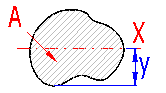### 2.10 List of materials.

In the pop-up list, select the material of the calculated beam. Structural steel with strength 36ksi and 50ksi is commonly available for ANSI profiles and structural steel EC 3, EN 10025; Fe 360; Fe 430; Fe 510 for DIN profiles .

• Limiting slenderness SRc (c) for the materials from the drop down menu is determined according to the equation SRc (lc)=(p2 * E / (sy*0.5))^0.5.
• The values given for wood are along the fibres (higher values).
• The beams can be delivered in a wide range of materials - depending on individual producers. Therefore, it is advisable to use information in catalogues and offers from individual producers.
• If you need to enter your own material parameters, enable the check mark button in the respective row [2.11 to 2.13] and enter the value in the respective field.

### 2.13 Limiting slenderness (plastic /elastic).

Limiting slenderness SRc (c) is an important parameter of a specific material, distinguishing the area of elastic and inelastic buckling as well as the use of relevant equations. It is, therefore, suitable to verify the parameter for the specific material. The recommended value is determined according to the equation: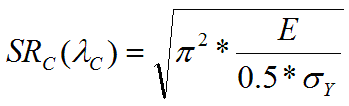Transfer the recommended value to proper cells by pressing the "<=" button

## Calculation and check of buckling. 

In this paragraph, you can design the beam profile able to transfer the desired load. You can also perform a check of a specific beam here.

#### Design:

For the design, set the beam length and force to be transferred by the beam [3.1, 3.2]. Select the safety coefficient and eccentricity degree [3.6, 3.7] and press the "Start" button. The program will select the minimum size of the selected type of profile or determine dimensions of the calculated profile. In case of the calculated profile, all profile dimensions are changed proportionally to the current values.

##### Example:

Rectangular profile with initial dimensions A=40mm, B=20mm will be adjusted e.g. to dimensions A=60mm, B=30mm after the calculation has been run

#### Check:

When checking a specific profile (dimensions have to be set in paragraph ), set beam length and force to be transferred by the beam [3.1, 3.2]. After setting the values, check the relevant safety coefficient [3.11, 3.15, 3.19, 3.27, 3.31]. A visual check can easily be performed in the diagram of critical stress on the slenderness rate. The current slenderness rate is marked with a red vertical line.

### 3.1 Actual strut length.

Set the actual length of the designed/checked strut.

Set the axial force affecting the strut.

### 3.3 Effective length.

The value is used in calculations. It is the actual length [3.1] multiplied by the reduced (effective) length coefficient [1.6].

### 3.4 Slenderness ratio.

The slenderness ratio of a specific beam determines which buckling zone the beam is in (simple compression, inflexible buckling, flexible buckling) and thus also the check method used for determination of the safety coefficient. The current slenderness ratio is marked with a vertical red line in the diagram.

### 3.5 Design of profile dimensions (Secant).

After the “Start” button has been pressed, the dimensions of the profile to accommodate the set conditions is calculated, on the basis of input data (strut mounting and length, type of profile, material, load, safety). The design is performed using the "Secant" method.

### 3.6 Safety coefficient.

The recommended values of the safety coefficient range from:

• Inflexible buckling zone - 3 to 8
• Flexible buckling zone - 5 to 10

### 3.7 Eccentricity ratio.

The degree of inaccuracy of a structure and load for the design of profile dimensions may be determined using this parameter. The parameter includes:

• Deviation of strut straightness
• Initial strut sag
• Origin of forces outside the strut axis
##### Recommended values:

0.25...steel structures
0.15...general engineering
0.05...accurate rigid mountings

### 3.8 Euler (elastic buckling).

This formula is valid for the zone of elastic buckling – the current slenderness rate [3.4] must be bigger than the "Critical (limiting) slenderness rate" [2.13].

### 3.12 Linear formula, Tetmajer.

Valid in the zone of inelastic buckling – the current slenderness rate [3.4] must be smaller than the "Critical (limiting) slenderness rate" [2.13].

### 3.16 Parabolic formula, Johnson.

Valid in the zone of inelastic buckling – the current slenderness rate [3.4] must be smaller than the "Critical (limiting) slenderness rate" [2.13].

### 3.20 Secant formula.

In cases when force does not act directly in the strut axis (mounting imperfections), or the strut is not accurately straight (manufacturing imperfections, sag), the so-called Secant formula is used to calculate stress in the extreme fibre of the profile.

### 3.21 Eccentricity.

Set eccentricity of a checked strut as shown in the picture. If the checkbox is checked, eccentricity is filled in automatically to achieve the eccentricity degree set in the drop down menu [3.23].see [3.7]

### 3.28 Simple compression.

Valid for short struts which are not subject to buckling.

### 3.32 Calculation of maximum force.

The maximum axial load of the strut for individual above-mentioned methods is calculated for the set safety coefficient [3.33]. If the checkbox is checked, the safety coefficient from line [3.6] is used.

## Setting calculations, change the language.

Information on setting of calculation parameters and setting of the language can be found in the document "Setting calculations, change the language".

## Workbook (calculation) modifications.

General information on how to modify and extend calculation workbooks is mentioned in the document "Workbook (calculation) modifications".